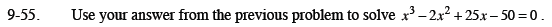Home > CCA2 > Chapter 9 > Lesson 9.2.1 > Problem9-55

9-55.

Use your answer from the previous problem to solve x3 − 2x2 + 25x − 50 = 0. Homework Help ✎$x^{3}-2x^{2}+25x-50=\left(x-2\right)\left(x^{2}+25\right)$

Use the Zero Product Property to solve.
This is a 3rd degree polynomial, so you need 3 solutions.